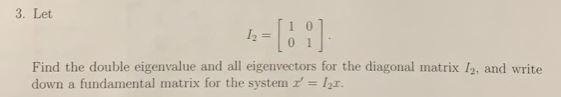3. Let12=101Find the double eigenvalue and all eigenvectors for the diagonal matrix I2, and writedown a fundamental matrix for the system' lr.

Question

Pls show me step by stephelp_outlineImage Transcriptionclose3. Let 12=101 Find the double eigenvalue and all eigenvectors for the diagonal matrix I2, and write down a fundamental matrix for the system' lr. fullscreen
Step 1
Step 2
Step 3

Want to see the full answer?

See Solution

Want to see this answer and more?

Our solutions are written by experts, many with advanced degrees, and available 24/7

See Solution
Tagged in

Math